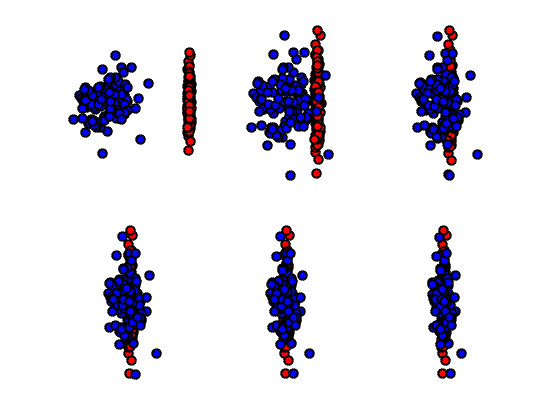$\newcommand{\NN}{\mathbb{N}} \newcommand{\CC}{\mathbb{C}} \newcommand{\GG}{\mathbb{G}} \newcommand{\LL}{\mathbb{L}} \newcommand{\PP}{\mathbb{P}} \newcommand{\QQ}{\mathbb{Q}} \newcommand{\RR}{\mathbb{R}} \newcommand{\VV}{\mathbb{V}} \newcommand{\ZZ}{\mathbb{Z}} \newcommand{\FF}{\mathbb{F}} \newcommand{\KK}{\mathbb{K}} \newcommand{\UU}{\mathbb{U}} \newcommand{\EE}{\mathbb{E}} \newcommand{\Aa}{\mathcal{A}} \newcommand{\Bb}{\mathcal{B}} \newcommand{\Cc}{\mathcal{C}} \newcommand{\Dd}{\mathcal{D}} \newcommand{\Ee}{\mathcal{E}} \newcommand{\Ff}{\mathcal{F}} \newcommand{\Gg}{\mathcal{G}} \newcommand{\Hh}{\mathcal{H}} \newcommand{\Ii}{\mathcal{I}} \newcommand{\Jj}{\mathcal{J}} \newcommand{\Kk}{\mathcal{K}} \newcommand{\Ll}{\mathcal{L}} \newcommand{\Mm}{\mathcal{M}} \newcommand{\Nn}{\mathcal{N}} \newcommand{\Oo}{\mathcal{O}} \newcommand{\Pp}{\mathcal{P}} \newcommand{\Qq}{\mathcal{Q}} \newcommand{\Rr}{\mathcal{R}} \newcommand{\Ss}{\mathcal{S}} \newcommand{\Tt}{\mathcal{T}} \newcommand{\Uu}{\mathcal{U}} \newcommand{\Vv}{\mathcal{V}} \newcommand{\Ww}{\mathcal{W}} \newcommand{\Xx}{\mathcal{X}} \newcommand{\Yy}{\mathcal{Y}} \newcommand{\Zz}{\mathcal{Z}} \newcommand{\al}{\alpha} \newcommand{\la}{\lambda} \newcommand{\ga}{\gamma} \newcommand{\Ga}{\Gamma} \newcommand{\La}{\Lambda} \newcommand{\Si}{\Sigma} \newcommand{\si}{\sigma} \newcommand{\be}{\beta} \newcommand{\de}{\delta} \newcommand{\De}{\Delta} \renewcommand{\phi}{\varphi} \renewcommand{\th}{\theta} \newcommand{\om}{\omega} \newcommand{\Om}{\Omega} \renewcommand{\epsilon}{\varepsilon} \newcommand{\Calpha}{\mathrm{C}^\al} \newcommand{\Cbeta}{\mathrm{C}^\be} \newcommand{\Cal}{\text{C}^\al} \newcommand{\Cdeux}{\text{C}^{2}} \newcommand{\Cun}{\text{C}^{1}} \newcommand{\Calt}{\text{C}^{#1}} \newcommand{\lun}{\ell^1} \newcommand{\ldeux}{\ell^2} \newcommand{\linf}{\ell^\infty} \newcommand{\ldeuxj}{{\ldeux_j}} \newcommand{\Lun}{\text{\upshape L}^1} \newcommand{\Ldeux}{\text{\upshape L}^2} \newcommand{\Lp}{\text{\upshape L}^p} \newcommand{\Lq}{\text{\upshape L}^q} \newcommand{\Linf}{\text{\upshape L}^\infty} \newcommand{\lzero}{\ell^0} \newcommand{\lp}{\ell^p} \renewcommand{\d}{\ins{d}} \newcommand{\Grad}{\text{Grad}} \newcommand{\grad}{\text{grad}} \renewcommand{\div}{\text{div}} \newcommand{\diag}{\text{diag}} \newcommand{\pd}{ \frac{ \partial #1}{\partial #2} } \newcommand{\pdd}{ \frac{ \partial^2 #1}{\partial #2^2} } \newcommand{\dotp}{\langle #1,\,#2\rangle} \newcommand{\norm}{|\!| #1 |\!|} \newcommand{\normi}{\norm{#1}_{\infty}} \newcommand{\normu}{\norm{#1}_{1}} \newcommand{\normz}{\norm{#1}_{0}} \newcommand{\abs}{\vert #1 \vert} \newcommand{\argmin}{\text{argmin}} \newcommand{\argmax}{\text{argmax}} \newcommand{\uargmin}{\underset{#1}{\argmin}\;} \newcommand{\uargmax}{\underset{#1}{\argmax}\;} \newcommand{\umin}{\underset{#1}{\min}\;} \newcommand{\umax}{\underset{#1}{\max}\;} \newcommand{\pa}{\left( #1 \right)} \newcommand{\choice}{ \left\{ \begin{array}{l} #1 \end{array} \right. } \newcommand{\enscond}{ \left\{ #1 \;:\; #2 \right\} } \newcommand{\qandq}{ \quad \text{and} \quad } \newcommand{\qqandqq}{ \qquad \text{and} \qquad } \newcommand{\qifq}{ \quad \text{if} \quad } \newcommand{\qqifqq}{ \qquad \text{if} \qquad } \newcommand{\qwhereq}{ \quad \text{where} \quad } \newcommand{\qqwhereqq}{ \qquad \text{where} \qquad } \newcommand{\qwithq}{ \quad \text{with} \quad } \newcommand{\qqwithqq}{ \qquad \text{with} \qquad } \newcommand{\qforq}{ \quad \text{for} \quad } \newcommand{\qqforqq}{ \qquad \text{for} \qquad } \newcommand{\qqsinceqq}{ \qquad \text{since} \qquad } \newcommand{\qsinceq}{ \quad \text{since} \quad } \newcommand{\qarrq}{\quad\Longrightarrow\quad} \newcommand{\qqarrqq}{\quad\Longrightarrow\quad} \newcommand{\qiffq}{\quad\Longleftrightarrow\quad} \newcommand{\qqiffqq}{\qquad\Longleftrightarrow\qquad} \newcommand{\qsubjq}{ \quad \text{subject to} \quad } \newcommand{\qqsubjqq}{ \qquad \text{subject to} \qquad } \newcommand{\eqdef}{\equiv}$

Advanced Topics on Sinkhorn Algorithm

# Advanced Topics on Sinkhorn Algorithm

This numerical tour explore several extensions of the basic Sinkhorn method.

## Installing toolboxes and setting up the path.

You need to download the following files: signal toolbox and general toolbox.

You need to unzip these toolboxes in your working directory, so that you have toolbox_signal and toolbox_general in your directory.

For Scilab user: you must replace the Matlab comment '%' by its Scilab counterpart '//'.

Recommandation: You should create a text file named for instance numericaltour.sce (in Scilab) or numericaltour.m (in Matlab) to write all the Scilab/Matlab command you want to execute. Then, simply run exec('numericaltour.sce'); (in Scilab) or numericaltour; (in Matlab) to run the commands.

Execute this line only if you are using Matlab.

getd = @(p)path(p,path); % scilab users must *not* execute this


Then you can add the toolboxes to the path.

getd('toolbox_signal/');
getd('toolbox_general/');

Warning: Name is nonexistent or not a directory:
/Users/gpeyre/Dropbox/github/numerical-tours/matlab/m_files/toolbox_signal


## Log-domain Sinkhorn

For simplicity, we consider uniform distributions on point clouds, so that the associated histograms are $$(a,b) \in \RR^n \times \RR^m$$ being constant $$1/n$$ and $$1/m$$.

n = 100;
m = 200;
d = 2; % Dimension of the clouds.
a = ones(n,1)/n;
b = ones(1,m)/m;


Point clouds $$x$$ and $$y$$.

x = rand(2,n)-.5;
theta = 2*pi*rand(1,m);
r = .8 + .2*rand(1,m);
y = [cos(theta).*r; sin(theta).*r];


Display of the two clouds.

plotp = @(x,col)plot(x(1,:)', x(2,:)', 'o', 'MarkerSize', 9, 'MarkerEdgeColor', 'k', 'MarkerFaceColor', col, 'LineWidth', 2);
clf; hold on;
plotp(x, 'b');
plotp(y, 'r');
axis('off'); axis('equal');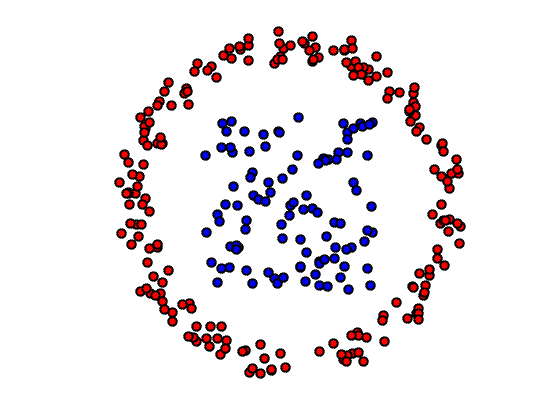Cost matrix $$C_{i,j} = \norm{x_i-y_j}^2$$.

distmat = @(x,y)sum(x.^2,1)' + sum(y.^2,1) - 2*x.'*y;
C = distmat(x,y);


Sinkhorn algorithm is originally implemented using matrix-vector multipliciation, which is unstable for small epsilon. Here we consider a log-domain implementation, which operates by iteratively updating so-called Kantorovitch dual potentials $$(f,g) \in \RR^n \times \RR^m$$.

The update are obtained by regularized c-transform, which reads $f_i \leftarrow {\min}_\epsilon^b( C_{i,\cdot} - g )$ $g_j \leftarrow {\min}_\epsilon^a( C_{\cdot,j} - f ),$ where the regularized minimum operator reads ${\min}_\epsilon^a(h) \eqdef -\epsilon \log \sum_i a_i e^{-h_i/\epsilon}.$

mina = @(H,epsilon)-epsilon*log( sum(a .* exp(-H/epsilon),1) );
minb = @(H,epsilon)-epsilon*log( sum(b .* exp(-H/epsilon),2) );


The regularized min operator defined this way is non-stable, but it can be stabilized using the celebrated log-sum-exp trick, wich relies on the fact that for any constant $$c \in \RR$$, one has ${\min}_\epsilon^a(h+c) = {\min}_\epsilon^a(h) + c,$ and stabilization is achieved using $$c=\min(h)$$.

mina = @(H,epsilon)mina(H-min(H,[],1),epsilon) + min(H,[],1);
minb = @(H,epsilon)minb(H-min(H,[],2),epsilon) + min(H,[],2);


Value of $$\epsilon$$.

epsilon = .01;


Exercice 1: (check the solution) Implement Sinkhorn in log domain.

exo1;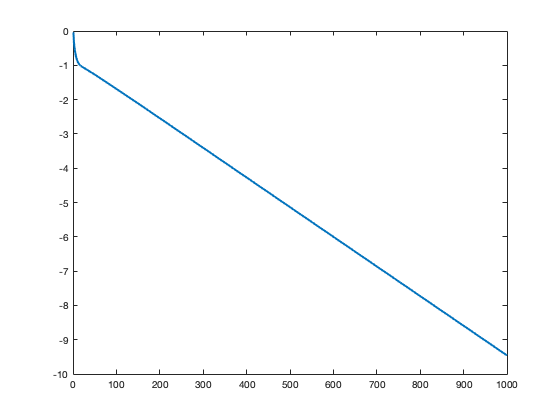Exercice 2: (check the solution) Study the impact of $$\epsilon$$ on the convergence rate of the algorithm.

exo2;


## Wasserstein Flow for Matching

We aim at performing a "Lagrangian" gradient (also called Wasserstein flow) descent of Wasserstein distance, in order to perform a non-parametric fitting. This corresponds to minimizing the energy function $\Ee(z) \eqdef W_\epsilon\pa{ \frac{1}{n}\sum_i \de_{z_i}, \frac{1}{m}\sum_i \de_{y_i} }.$

Here we have denoted the Sinkhorn score as $W_\epsilon(\al,\be) \eqdef \dotp{P}{C} - \epsilon \text{KL}(P|ab^\top)$ where $$\al=\frac{1}{n}\sum_i \de_{x_i}$$ and $$\be=\frac{1}{m}\sum_i \de_{y_i}$$ are the measures (beware that $$C$$ depends on the points positions).

z = x; % initialization


The gradient of this energy reads $( \nabla \Ee(z) )_i = \sum_j P_{i,j}(z_i-y_j) = a_i z_i - \sum_j P_{i,j} y_j,$ where $$P$$ is the optimal coupling. It is better to consider a renormalized gradient, which corresponds to using the inner product associated to the measure $$a$$ on the deformation field, in which case $( \bar\nabla \Ee(z) )_i = z_i - \bar y_i \qwhereq \bar y_i \eqdef \frac{\sum_j P_{i,j} y_j}{a_i}.$ Here $$\bar y_i$$ is often called the "barycentric projection" associated to the coupling matrix $$P$$.

First run Sinkhorn, beware you need to recompute the cost matrix at each step.

epsilon = .01;
niter = 300;
C = distmat(z,y);
for it=1:niter
g = mina(C-f,epsilon);
f = minb(C-g,epsilon);
end
P = a .* exp((f+g-C)/epsilon) .* b;


G = z - ( y*P' ) ./ a';


Display the gradient field.

clf; hold on;
plotp(x, 'b');
plotp(y, 'r');
for i=1:n
plot([x(1,i), x(1,i)-G(1,i)], [x(2,i), x(2,i)-G(2,i)], 'k');
end
axis('off'); axis('equal');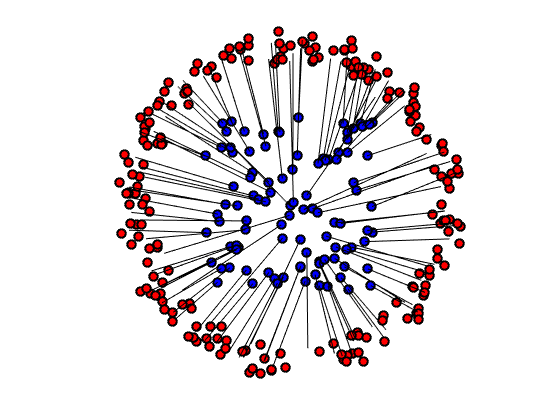Set the descent step size.

tau = .1;


Update the point cloud.

z = z - tau * G;


Exercice 3: (check the solution) Implement the gradient flow.

exo3;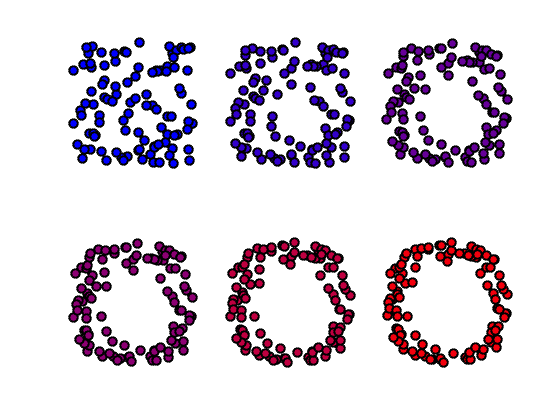Exercice 4: (check the solution) Show the evolution of the fit as $$\epsilon$$ increases. What do you observe. Replace the Sinkhorn score $$W_\epsilon(\al,\be)$$ by the Sinkhorn divergence $$W_\epsilon(\al,\be)-W_\epsilon(\al,\al)/2-W_\epsilon(\be,\be)/2$$.

exo4;


## Generative Model Fitting

The Wasserstein is a non-parametric idealization which does not corresponds to any practical application. We consider here a simple toy example of density fitting, where the goal is to find a parameter $$\theta$$ to fit a deformed point cloud of the form $$(g_\theta(x_i))_i$$ using a Sinkhorn cost. This is ofen called a generative model in the machine learning litterature, and corresponds to the problem of shape registration in imaging.

The matching is achieved by solving $\min_\th \Ff(\th) \eqdef \Ee(G_\th(z)) = W_\epsilon\pa{ \frac{1}{n}\sum_i \de_{g_\th(z_i)}, \frac{1}{m}\sum_i \de_{y_i} },$ where the function $$G_\th(z)=( g_\th(z_i) )_i$$ operates independently on each point.

The gradient reads $\nabla \Ff(\th) = \sum_i \partial g_\th(z_i)^*[ \nabla \Ee(G_\th(z))_i ],$ where $$\partial g_\th(z_i)^*$$ is the adjoint of the Jacobian of $$g_\th$$.

We consider here a simple model of affine transformation, where $$\th=(A,h) \in \RR^{d \times d} \times \RR^d$$ and $$g_\th(z_i)=Az_i+h$$.

Denoting $$v_i = \nabla \Ee(G_\th(z))_i$$ the gradient of the Sinkhorn loss (which is computed as in the previous section), the gradient with respect to the parameter reads $\nabla_A \Ff(\th) = \sum_i v_i z_i^\top \qandq \nabla_h \Ff(\th) = \sum_i v_i.$

Generate the data.

z = randn(2,n)*.2;
y = randn(2,m)*.2; y(1,:) = y(1,:)*.05 + 1;


Initialize the parameters.

A = eye(2); h = zeros(2,1);


Display the clouds.

clf; hold on;
plotp(A*z+h, 'b'); plotp(y, 'r');
axis('off'); axis('equal');Compute the gradient with respect to parameters.

x = A*z+h;
C = distmat(x,y);
f = zeros(n,1);
for it=1:niter
g = mina(C-f,epsilon);
f = minb(C-g,epsilon);
end
P = a .* exp((f+g-C)/epsilon) .* b;
% gradient with respect to positions
v = a' .* z - ( y*P' );
% gradient with respect to parameters
nabla_A = v*z';
nabla_h = sum(v,2);


Exercice 5: (check the solution) Implement the gradient descent.

exo5;